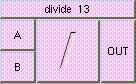DIVIDETHE BOX'S MAIN HELP: This is the division function. The result is the quotient of A / B. A and B may be scalar, vector, or colors. The result will be a scalar if both A and B are scalars. The result will be a vector if either A and B are vectors. The result will be a color if either A and B are colors. A type mis-match will result from the division of colors and vectors. A (input): This is the value to be divided, the dividend. The input may be a scalar, vector, or color. B (input): This is the divisor. The input may be a scalar, vector, or color. OUT (output): This is the division function. The result is the quotient of A / B. A and B may be scalar, vector, or colors. The result will be a scalar if both A and B are scalars. The result will be a vector if either A and B are vectors. The result will be a color if either A and B are colors. A type mis-match will result from the division of colors and vectors.

<-- BACK TO Math